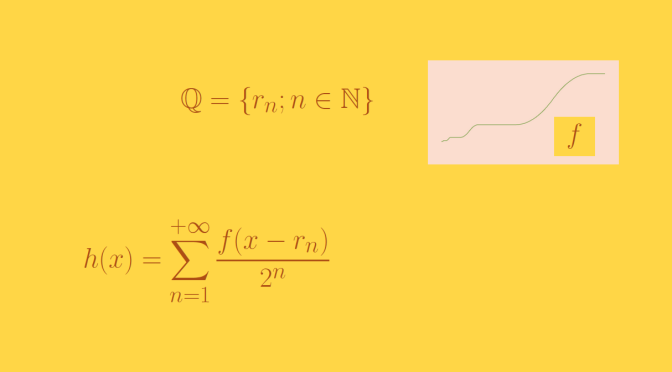# A continuous function not differentiable at the rationals but differentiable elsewhere

We build here a continuous function of one real variable whose derivative exists on $$\mathbb{R} \setminus \mathbb{Q}$$ and doesn’t have a left or right derivative on each point of $$\mathbb{Q}$$.

As $$\mathbb{Q}$$ is (infinitely) countable, we can find a bijection $$n \mapsto r_n$$ from $$\mathbb{N}$$ to $$\mathbb{Q}$$. We now reuse the function $$f$$ defined here. Recall $$f$$ main properties:

PR1
$$f$$ is continuous on $$\mathbb{R}$$.
PR2
$$f$$ has a derivative $$g$$ on $$\mathbb{R}^*$$ and $$\vert g(x) \vert \le 1$$ for $$x \in \mathbb{R}$$.
PR3
$$\vert f(x) \vert \le \frac{1}{3}$$ for $$x \in \mathbb{R}$$.
PR4
Therefore $$f$$ is Lipschitz continuous and $$1$$ is a Lipschitz constant for $$f$$, i.e. $$f$$ is a short map.

For $$n \in \mathbb{N}$$ we define $$\displaystyle u_n(x)=\frac{f(x-r_n)}{2^n}$$ and $$\displaystyle h_n(x)=\sum_{k=0}^n u_n(x)$$. According to PR1 those functions are defined on $$\mathbb{R}$$ and continuous. Let’s now consider the function series:
$\begin{array}{l|rcl} h : & \mathbb{R} & \longrightarrow & \mathbb{R} \\ & x & \longmapsto & \displaystyle \sum_{k=0}^{+\infty} u_n(x) \end{array}$ $$h$$ is defined on $$\mathbb{R}$$ due to PR2. PR2 also implies that $$h$$ is normally convergent. Therefore $$h$$ is continuous on $$\mathbb{R}$$ as the $$u_n$$ are continuous.

If $$a \in \mathbb{R} \setminus \mathbb{Q}$$ we have $$a-r_n \neq 0$$ for all $$n \in \mathbb{N}$$ and according to PR3 $$u_n$$ is differentiable at $$a$$ with:
$u_n^{\prime}(a)=\frac{f^{\prime}(a-r_n)}{2^n}=\frac{g(a-r_n)}{2^n}$ $$h_n$$ which is a finite sum of functions differentiable at $$a$$ is also differentiable at $$a$$.

The inequality $$\vert g(x) \vert \le 1$$ valid for $$x \in \mathbb{R}$$ ensures that $$\displaystyle \sum_{n=0}^{+\infty} u_n^{\prime}(a)$$ converges. We denote $$H(a)$$ the sum of that series, $$H_n(a)=h_n^{\prime}(a)$$ and
$D(x)=\frac{h(x)-h(a)}{x-a} \mbox{ and } D_n(x)=\frac{h_n(x)-h_n(a)}{x-a}$ For $$p,q \in\mathbb{N}$$ and $$x \neq a$$ the following inequalities hold:
\begin{aligned} \vert (h_q(x)-h_q(a))-(h_q(x)-h_q(a)) \vert &\le \sum_{n=p+1}^q \frac{\vert f(x-r_n)-f(a-r_n)\vert}{2^n}\\ &\le \sum_{n=p+1}^q \frac{\vert x – a \vert}{2^n} \le \frac{\vert x – a\vert}{2^p} \end{aligned} Therefore $$\vert D_q(x) -D_p(x) \vert \le \frac{1}{2^p}$$. For $$\epsilon > 0$$, we pick up $$p \in \mathbb{N}$$ in order to have $$\frac{1}{2^p} \le \frac{\epsilon}{3}$$ and $$\vert H_p(a) – H(a) \vert \le \frac{\epsilon}{3}$$, which is possible as $$\lim\limits_{n \to +\infty} H_n(a)=H(a)$$. $$h_p$$ is differentiable at $$a$$ and $$h^\prime_p(a)=H_p(a)$$. So we can find $$\alpha > 0$$ in order to have $$\vert D_p(x)-H_p(a) \vert \le \frac{\epsilon}{3}$$ when $$\vert x-a \vert < \alpha$$ and $$x \neq a$$. For $$x$$ satisfying those last conditions we then have: \begin{aligned}\vert D(x)-H(a) \vert &\le \vert D(x) - D_p(x) \vert + \vert D_p(x) - H_p(a) \vert\\ &+ \vert H_p(a) - H(a) \vert \le \epsilon \end{aligned} This proves that $$h$$ is differentiable at $$a$$ with $$h^\prime(a) = \lim\limits_{n \to +\infty} h_n^\prime(a)$$. For $$a \in \mathbb{Q}$$, we can find $$p \in \mathbb{N}$$ with $$a = r_p$$. Following a similar proof than above, the function $$l_p: x \mapsto h(x) - u_p(x)$$ is differentiable at $$a$$. As $$f$$ does not have left and right derivatives at $$0$$, $$u_p$$ does not have left and right derivatives at $$a$$. finally, the equality $$h=l_p+u_p$$ implies that $$h$$ also does not have left and right derivatives at $$a$$. Conclusion: the function $$h$$ is differentiable at all irrational points but does not have left or right derivative at all rational points.